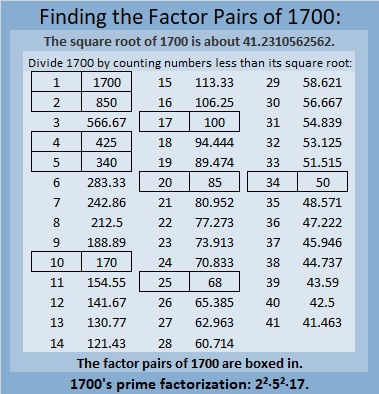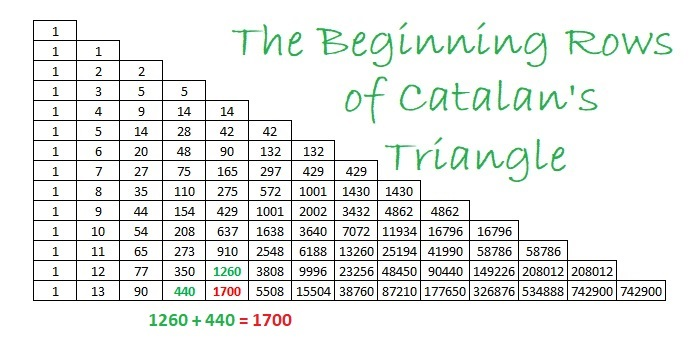# 1700 Time for a Horse Race!

### Today’s Puzzle:

I’ve made a table of all the numbers from 1601 to 1700, their prime factorizations, and how many factors each of those numbers has.Each number from 1601 to 1700 has 2, 3, 4, 6, 8, 10, 12, 16, 18, 24, 30 or 40 factors. Do more numbers have 2 factors, 3 factors, or a different number? Sure, you could use the table to count, but it will be more fun if we make a horse race out of it. What pony will you pick, 2, 3, 4, 6? Make your prediction of the winner, and then watch the horse race to see if you were right.

Here are all the horses lined up at the gate. Pick your pony!And they’re off!make science GIFs like this at MakeaGif
The first half of the race was VERY interesting to me. Three different horses held the lead, and the leaders in the race was even neck and neck some of the time. After you know the winner, watch the race again, following the winner from start to finish.

### Factors of the Number 1700:

• 1700 is a composite number.
• Prime factorization: 1700 = 2 × 2 × 5 × 5 × 17, which can be written 1700 = 2² × 5² × 17.
• 1700 has at least one exponent greater than 1 in its prime factorization so √1700 can be simplified. Taking the factor pair from the factor pair table below with the largest square number factor, we get √1700 = (√100)(√17) = 10√17.
• The exponents in the prime factorization are 2, 2, and 1. Adding one to each exponent and multiplying we get (2 + 1)(2 + 1)(1 + 1) = 3 × 3 × 2 = 18. Therefore 1700 has exactly 18 factors.
• The factors of 1700 are outlined with their factor pair partners in the graphic below.### More About the Number 1700:

1700 is the sum of two squares THREE different ways:
40² + 10² = 1700,
38² + 16² = 1700, and
32² + 26² = 1700.

Those sum of two squares mean 1700 is the hypotenuse of some Pythagorean triples, SEVEN to be exact:

1. 260-1680-1700, which is 20 times (13-84-85),
2. 348-1664-1700, which is 4 times (87-416-425), but it can also be calculated from 32² – 26², 2(32)(26), 32² + 26²
3. 476-1632-1700, which is (7-24-25) times 68,
4. 720-1540-1700, which is 20 times (36-77-85),
5. 800-1500-1700, which is (8-15-17) times 100, but it can also be calculated from 2(40)(10), 40² – 10², 40² + 10²,
6. 1020-1360-1700, which is (3-4-5) times 340,
7. 1188-1216-1700, which is 4 times (297-304-425), but it can also be calculated from 38² – 16², 2(38)(16), 38² + 16².

As OEIS.org informs us, 1700 is a Catalan number. It is found in the C(13,4) position, as shown below. With the exception of the lone 1 in the 0th row of the triangle, every number in Catalan’s triangle is the sum of the number above it and the number to its left. For example, 1700 = 1260 + 440.I hope you have enjoyed learning about the number 1700.